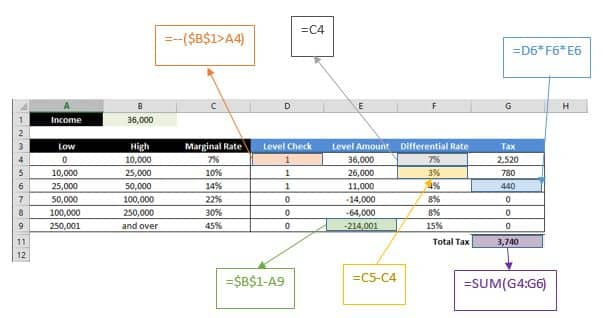It’s that time of the year! Of course there are all kinds of tools out there you can use to calculate and submit your taxes for the last year, but it’s always a good idea to double check everything and if you prefer to do everything by hand, you might find this guide even more useful.

The tax brackets can be confusing to work with. Different ‘levels’ of your income needs to be multiplied with a different percentage to find the total. This can be done with the conventional Excel formulas like IF and a bunch of others, but that’s the long and hard to read approach. Excel has a function that is actually perfect for this job: SUMPRODUCT.

We should add a disclaimer here. We are not a financial consultant company and we do not provide any assistance for tax or personal finance purposes. The methods you will see in this article should only give you ideas as to how you can build similar tools on your own. The data used in this article was retrieved at the of its publishing and may not reflect the current rates.

You can download the workbook we’re going to be using by pressing the button below.

## Basics

The United States tax system follows a structure that is similar to what is used by most countries. There are income brackets, and you pay your taxes based on what you made the previous year. Of course we’re not going to go too much into the details of the tax system, but this can help understand how to utilize Excel for calculations.

 Low High Marginal Rate 0 10,000 7% 10,000 25,000 10% 25,000 50,000 14% 50,000 100,000 22% 100,000 250,000 30% 250,000 and over 45%

What is Marginal Rate? Let’s take an example from this table. Say your income is \$36,000 per year. This means that you owe the government,

• 36,000 * 7% +
• 26,000 * 3% +
• 11,000 * 4%

In total this makes \$3,740. Let’s see how we got to this number.

We used differential income levels and rates for this calculation. First, we multiplied the total income of \$36,000 by 7%, because all income, (even if you made 1\$) is taxed by at least 7% of the total.

Next, we used the amount that exceeds what we used in the first step (\$10,000). The difference comes to \$26,000 (36,000-10,000) and must be multiplied by 3% which is the difference between the second bracket rate (10%). We essentially added the missing 7% of this step in the previous one.

Finally, we use all of \$36,000 because it is less than next step’s lower limit of \$50,000. Now we multiply the remaining \$11,000 (36,000-25,000) by 7% (22%-14%) and that makes \$3,740. Let’s illustrate these calculations on a table.

 Low High Marginal Rate Level Amount Differential Rate Tax 0 10,000 7% 36,000 7% 2,520 10,000 25,000 10% 26,000 3% 780 25,000 50,000 14% 11,000 4% 440 50,000 100,000 22% -14,000 8% -1,120 100,000 250,000 30% -64,000 8% -5,120 250,001 and over 45% -214,001 15% -32,100 Total Tax 3,740

The numbers in the tax column are not exactly what we found before! And what about the negative numbers? Well, Excel can’t know where our income bracket level ends. Therefore, we must use this method to be able to find the total amount owed, regardless of the entered amount. This way, \$36,000 will stop at the third bracket. To get rid of negative values, we need another ‘helper’ column to check whether our income is within a given limit. Here is how we built this table in Excel,Double-minus (--) converts logical values to numbers (TRUE to 1 and FALSE to 0). We converted the logical values into numbers so that we can use them easily in other calculations. It’s all pretty nice, but there’s actually a better way to do this, enter SUMPRODCUT.

## SUMPRODUCT

The SUMPRODUCT function multiplies the corresponding values in selected arrays, and returns the sum. There are other ways to do this, like using nested IF statements or other formulas. But the SUMPRODUCT function can operate multiple cells at once and this means that you can create such tables very quickly using only one formula. The SUMPRODUCT function can function like an array formula by pressing Ctrl+Alt+Enter and this behavior is what we need to simplify the tax calculation process. Formula syntax is very straightforward,

SUMPRODUCT(array1, [array2], [array3], ...)

## Using the SUMPRODUCT Formula

We’re going to need to check for entered amount, bracket level, and differential tax rates for arguments of the SUMPRODUCT. Because we don’t need ‘helper’ columns anymore, we’re going to use ranges instead.

• Check value level: from =--(\$B\$1>A4) to =--(\$B\$1>A6:A11)
• Check bracket level: from =\$B\$1-A4 to =(\$B\$1-A6:A11)
• Differential tax rates: from =C5-C4 to (C6:C11-C5:C10) *

* Note: We used “=C4” to get first bracket rate as differential rate because it is the first value, and there is no need to subtract 0 from 7%. However, to keep everything in a single formula, we added a blank dummy row (C5) which is evaluated as 0. This means that the differential tax rate calculation of arrays starts at C6-C5.

Here is the formula,

=SUMPRODUCT(--(\$B\$1>A6:A11),(\$B\$1-A6:A11),(C6:C11-C5:C10))## Actual Rates

If you don’t need a table structure you can use the formulas below with Federal Tax Rates of 2017 to find the total tax. All you need to do is to copy the formula and paste it your Excel worksheet, anywhere except cell B1. And enter your income into B1.

• Single:

=SUMPRODUCT(--(\$B\$1>{0;9325;37950;91900;191650;416700;418400}),(\$B\$1-{0;9325;37950;91900;191650;416700;418400}),{0.1;0.05;0.1;0.03;0.05;0.02;0.046})

• Married Filling Joint:

=SUMPRODUCT(--(\$B\$1>{0;18650;75900;153100;233350;416700;470700}),(\$B\$1-{0;18650;75900;153100;233350;416700;470700}),{0.1;0.05;0.1;0.03;0.05;0.02;0.046})